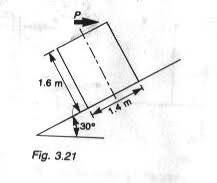# Sliding/toppling of a block on a ramp

1. A uniformly loaded crate of weight 1000N is held at rest on an inclined place. A gradually increasing force P is applied horizontally as shown. If coefficient of friction, u, = 0.2, determine whether the crate first slides or topples and the least force required3. Reaction R = 1000 cos 30 + P sin 30
F = uR
P cos 30 - F -mg sin 30 = 0

tiny-tim
Homework Helper
Welcome to PF!

Welcome to PF!(have a mu: µ)
3. Reaction R = 1000 cos 30 + P sin 30
F = uR
P cos 30 - F -mg sin 30 = 0

Yes, that looks ok for the sliding equation …

now you need a toppling equation …

Hint: it will start to topple when the reaction force is at the corner, so take momentsAccording to the way i've worked it out... P comes out to be 697 N.. while the book's answer is 726 N.. this is stressing me out.. I can't figure out where's the mistake..

For the toppling bit, i guess i'll have to do a moment balance about the corner.. and if P < P for the sliding bit, then the block topples.. am i right?

tiny-tim
Homework Helper
For the toppling bit, i guess i'll have to do a moment balance about the corner.. and if P < P for the sliding bit, then the block topples.. am i right?

Yes.(but you can do a moment balance about any point … it makes no difference … just choose the point which you think will involve the least amount of arithmetic)
According to the way i've worked it out... P comes out to be 697 N.. while the book's answer is 726 N.. this is stressing me out.. I can't figure out where's the mistake..

Show us your full calculations, and then we can see what went wrong, and we'll know how to help!I found P to be 697 N.. which does not coincide with that of the book.. its stressing me out :s

Alright will do that...

∑V = 0
R = 1000 cos 30 + P sin 30
= 866 + 0.5 P

F = µR
= 0.2 ( 866 + 0.5P)
= 173.2 + 0.1P

∑H = 0
P cos 30 = F + 1000 sin 30
= 173.2 + 0.1P + 500

P = 673.1/(0.1 + cos 30)
= 697N

Last edited:
I found P to be 697 N.. which does not coincide with that of the book.. its stressing me out :s

tiny-tim
Homework Helper
∑H = 0
P cos 30 = F + 1000 sin 30
= 173.2 + 0.1P + 500

P = 673.1/(0.1 + cos 30)

Nope … (-0.1 + cos 30)Nope … (-0.1 + cos 30)Thanks loads! You saved my day ;) OOps now P is coming to 878 N :s

tiny-tim
Homework Helper
Thanks loads! You saved my day ;) OOps now P is coming to 878 N :s

Don't panic!

it's probably the other P, thenAh i give up.. Thanks for your kind help sir :)

tiny-tim
Homework Helper
Ah i give up.. Thanks for your kind help sir :)

But you haven't done the moment equation yet …

that'll probably give you a P that's less than 697NAttempting a moment balance about far right corner :p

(P cos 30 x 1.6) = (1000 cos 30 x 0.7) + ( P sin 30 x 0.7) + ( 1000 sin 30 x 0.8)
P = 971 N .. doesnt sound right

I get P = 753 for the minimum tipping force. I don't think the answer in your book is right.
Let x=1.4, y=1.6, w=1000N. Then the torque = (W/2) (x sin 30 + y cos 30) - P y cos 30.
This gives P = (W/2) ( x/y tan 30 +1) = 753 for zero torque.

tiny-tim
Homework Helper
Attempting a moment balance about far right corner :p

(P cos 30 x 1.6) = (1000 cos 30 x 0.7) + ( P sin 30 x 0.7) + ( 1000 sin 30 x 0.8)
P = 971 N .. doesnt sound right

can't follow thisyou need the perpendicular distance from the corner to the line of the external force to equal the perpendicular distance from the corner to the vertical line through the centre of mass (because both the friction force and the tipping-normal force have zero moment).

Try again.can't follow thisyou need the perpendicular distance from the corner to the line of the external force to equal the perpendicular distance from the corner to the vertical line through the centre of mass (because both the friction force and the tipping-normal force have zero moment).

Try again.(P cos 30 x 1.6) = (1000 cos 30 x 0.7) + (1000 sin 30 x 0.8)
P = 726 N!!! Wheee!! At last!.. .. thanks a lot for the walk through! Ah i'm not really a lumiere when it comes to mechanics.. You're an Ace!!

Last edited: